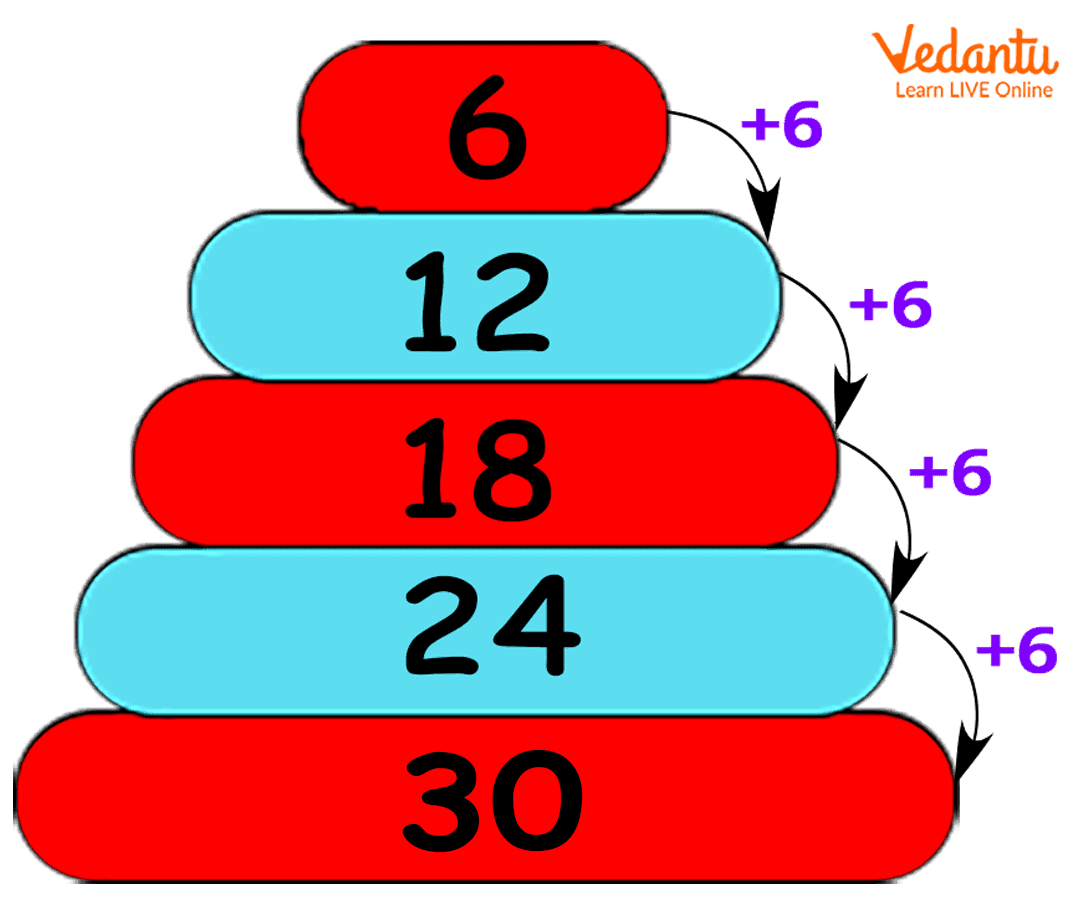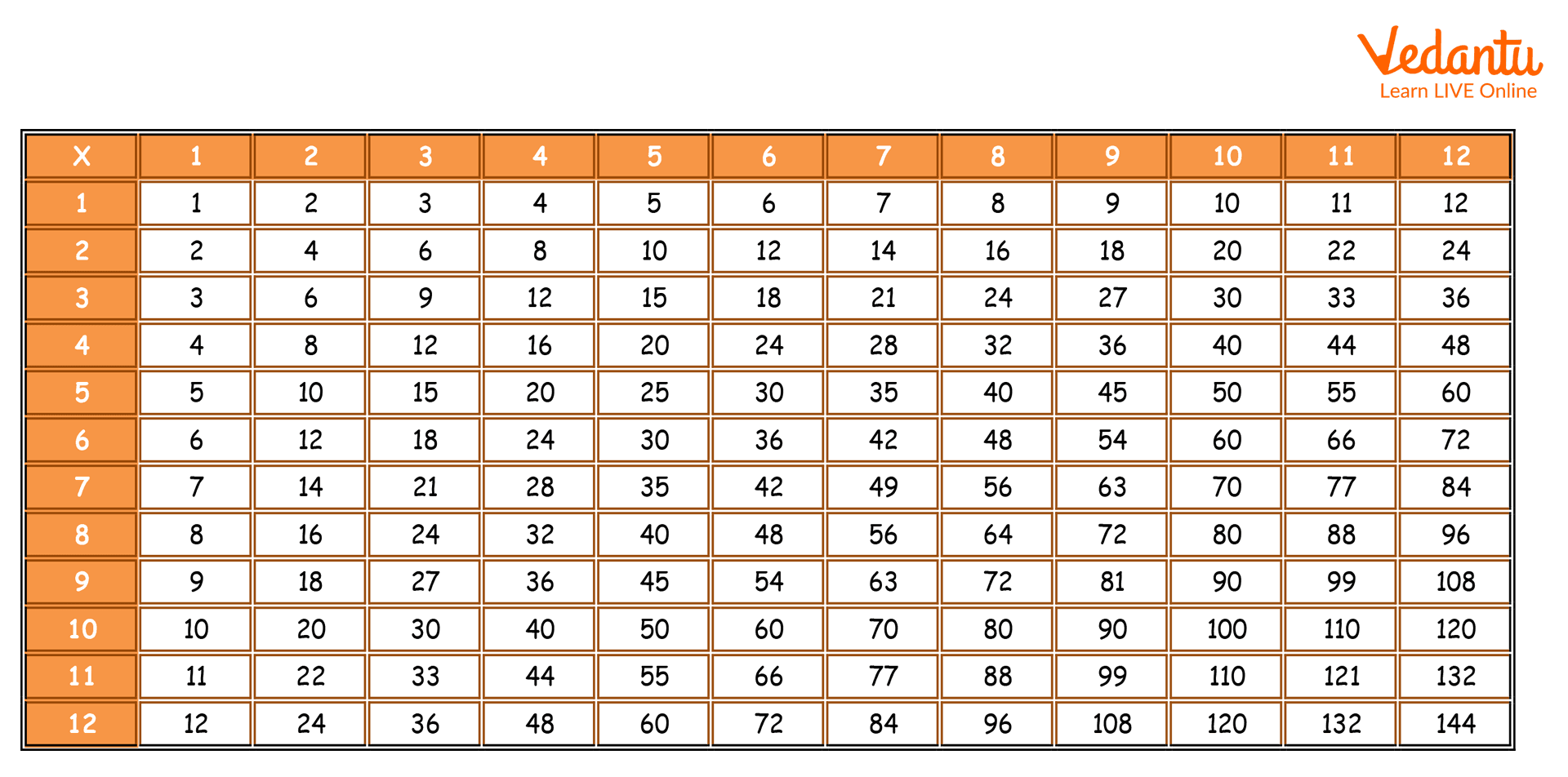Courses
Courses for Kids
Free study material
Offline Centres
More

# Multiples - Definitions, Explanation and ExamplesLast updated date: 07th Dec 2023
Total views: 315.9k
Views today: 7.15k## Introduction to Multiples

Being one of the most exciting chapters of mathematics, Multiples and Factors enables students to get into the fundamentals of calculating and simplifying equations. The chapter establishes a base for students which they can make use of while understanding more difficult areas of this subject. The chapter mainly contains the definition of multiples, how these are important in Mathematics, activity based on common factors and common multiples, etc., along with some examples.

The chapter also contains a detailed description and usage of factors and the differences between common factors and common multiples. These two are vital concepts related to each other; therefore, students should go through both these topics simultaneously to better understand them.

Multiples are an easy way of saying ‘manifold’ or a result of adding something multiple times. It is important that kids learn the meaning and uses of multiples in Maths because this is a concept that will be used in life “multiple times”! So let us learn more about this term.

## Definition of Multiple

The term multiple can be defined as the product of a number that is multiplied by another number. A number multiplied by different numbers will result in different multiples. Therefore, a multiple of a number can be reached by multiplying the number by a specific number or by adding the number to itself for a specific number of times.

### Examples of Multiples

1. The number 56 is a multiple of 7. Here, adding 7 eight times will result in the number 56. In other words, multiplying the integer 7 by 8 will give 56. It must be noted here that 56 is also a multiple of 8 since 8 added seven times will give 56 or 8 multiplied by 7 will result in 56.

1. Here is a table to list a few multiples of 4:

4 x 1 = 4

4 x 2 = 8

4 x 3 = 12

4 x 4 = 16

4 x 5 = 20

4 x 6 = 24

Therefore, it can be said that 4, 8, 12, 16, 20, 24 are all multiples of 4.Multiples of 6 by adding 6

### Games to Learn Multiples

There is an amazing game which you can play while travelling to school or some other place. This is called the Number plate Game, where you will need to call out the multiples of the first two numbers on the number plate of the vehicle ahead of you. It is a fun way to brush up on your counting skills and become a master of multiples.Multiplication table from 1 to 12

### What is a Factor?

Factors can be specified as whole numbers that can evenly divide another number. Precisely, when a number is considered as a factor of another second number, the first one has to divide the second number completely without keeping any remainder behind.

In other words, if the dividend is evenly divisible by a number or also called as a divisor, that particular divisor will be known as a factor of that dividend. Every number is supposed to have a common factor, such as one (1) and also the number itself. The highest common factor and lowest common multiple TNPSC questions generally include questions related to these; therefore, students need to go through a few examples to comprehend them.

For instance,

4 is considered as a factor of 24, as it divides 24 evenly and leaves 6 as quotient and zero (0) as remainder. On the other hand, 6 is also considered as the factor of 24, which leaves 4 as quotient and zero (0) as remainder.

Consequently, it is apparent that 24 has multiple factors, such as 1, 24, 4, 6, and 2, 3, 8, including 12, since all these numbers can divide 24 evenly without leaving any remainder.

As discussed earlier, the factor is a number that generally keeps no remainder behind after dividing a particular number. On the other hand, multiple is a number obtained by multiplying a whole number by some other numbers. Following is a list of key differences between common factors and multiples class 4:

## Difference Between Common Factors and Common Multiples

 Comparison Factor Multiples Factors Definition Multiples are a result, obtained when a whole number gets multiplied by another number. Factors can be referred to as an exact divisor of a specific number. Outcome It has to be equal or greater than a particular number. It can be less than or similar (equal) to a particular number. Number of factors/ multiples Infinite Finite Operation used Multiplication Division

Along with these differences, students should also be aware of the process of common factors and common multiples to compare them. Mathematics mainly deals with numbers and different operations, and factors and multiples are one of those kinds. The concepts are pretty basic and should be grasped properly to understand higher-level mathematics in a better way.

Furthermore, with assistance from e-learning platforms like Vedantu, one can elevate their knowledge of multiples. The model exam question papers, expert notes, along with online classes, and doubt clearing sessions, students can better their final exam preparations.

### Did You Know?

0 is a multiple of all numbers since any number multiplied by 0 will result in 0!

### Conclusion

Multiples are an important part of Maths and the topic should not be avoided or kept for later. It is an integral part of learning counting and calculation, and it is advisable to memorise the multiplication tables at least till 12.

## FAQs on Multiples - Definitions, Explanation and Examples

1. What are Prime Numbers?

Ans. When natural numbers consist of two factors such as 1 and the integer itself, those numbers are called a prime number. For example, 2 can be considered as a prime number as it also has two factors such as 1 and 2. On the contrary, the factors of number 6 will be 1, 2, 3, and 6. Therefore, it cannot be considered a prime number but a composite number for multiple factors.

One should remember that 1 can be a natural number factor; however, it cannot be considered a prime or composite number. In order to understand this concept better, students can also make use of co-prime numbers highest common factor and lowest common multiple NCERT for further information.

2. What do you Mean by Factor Pairs?

Ans. When two numbers get multiplied together to obtain a specific result or product, those numbers are called factor pairs. For instance, in order to get the product of 8, the multiplication process can look like: 2 x 4 and 1 x 8, in that case, the factor pairs for 8 have to be 2, 4, and 1, 8.

Most often, factors are given as pairs of numbers, and by multiplying them together, you get the original number. There are highest common factor and lowest common multiple calculators that students can use to calculate factor pairs or find out the highest and lowest among them.

3. Are the Highest Common Factors and Lowest Common Multiple Questions Available Online?

Ans. Factors and Multiples chapter comprises two key concepts that should be studied together to understand the basics and further dive deeper. Even though the concepts are easily comprehensible, students should also refer to various study materials where the questions and answers are available to help them. From detailed definitions to finding the least common multiple and highest common factor, key differences between both factors and multiples including, examples are easily accessible from renowned education sites like Vedantu.

The explanations of factors and multiples might vary; however, students can easily compare and find the most straightforward explanation to quickly understand the topic. They can also find common factors and common multiples class 6 worksheets in these websites and an online calculator to practice numerical.

4. Why is it necessary to learn multiples?

Multiples are the building blocks of basic mathematical computation and parents should encourage their children to pick up the multiplication tables as spontaneously as they would in case of having food. A ready knowledge of multiples will help children in solving a lot of things really fast.

5. What are some multiples of 12?

Some of the consecutive multiples of 12 are 12, 24, 36, 48, 60, 72, 84, 96, 108, 120, 144, and so on.